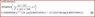# Maple issue - inverse laplace transform equation from a basic series RLC circuit

• Maple
• FOIWATER
In summary, the laplace equation from a basic series RLC circuit can be solved in the time domain, but requires the value for v(0) to be known. When the capacitor is charging, the same laplace equation must be used, but with the addition of a rise in voltage.

#### FOIWATER

Gold Member
maple issue -- inverse laplace transform equation from a basic series RLC circuit

Pretty simple for some of you I know, but I have a laplace transform equation from a basic series RLC circuit

((sV-v(0))/L+si(0))/(s^2+sR/L+1/LC) = I(s)

I want to take the inverse laplace of it, I am given all initial conditions, and constants for R,L, and C.

I've done laplace but cannot figure out how to take the inverse of this one, and cannot figure how to check it with maple..

Any help appreciated.

EDIT: initial conditions are L = 10H, v = 10v C = 1000uF i(0) = 1A R = 100ohms

Can I just fill in values at this point and attain 2s-.5/s^2+10s+100 and simplify this and bring it back to the time domain?

Sorry, I am asked for the current response in the time domain.. So I think this is the right approach... maybe I have made a mistake.

Any help with the maple would still be appreciated greatly. I am new to the program.

I get 2e^-5tcos(sqrt75)t - (21/2(sqrt75))e^-5tsin(sqrt75)t

I was expecting a cleaner result...

Last edited:

FOIWATER said:
Pretty simple for some of you I know, but I have a laplace transform equation from a basic series RLC circuit

((sV-v(0))/L+si(0))/(s^2+sR/L+1/LC) = I(s)

... Can I just fill in values at this point and attain 2s-.5/s^2+10s+100 and simplify this and bring it back to the time domain?

$$I(s) = \frac{2s-0.5}{s^2+10s+100}$$
I haven't checked your substitutions, but assuming the above is correct, then you should complete the square on denominator before attempting to take the inverse Laplace transform.

In general you would either factorize or complete the square. In this case it's easy to show that the denominator has no real roots, so completing the square is more appropriate.

Yes, and thankyou for the response.

I did complete the square, and separate the expression taking two inverse laplace transforms, I did not show the work.

I actually also got it figured out in maple, and received the correct answer by hand. The one up there is not correct, but it is close.

The correct solution is -4.5/sqrt75sinsqrt75t + cossqrt75t

I am having a larger issue now, when the capacitor is charged before t=0, and wether or not I can use the same laplace transforms for a capacitor that is now supplying power.

It was my initial response to simply put it on the other side of my kirchhoffs voltage equation, as a rise. However, I am not receiving correct results.

My professor adds a source in series to his capacitors and inductances, rather than simply laplace them.. he laplaces them seperately.

I am at wits end !

Last edited:

FOIWATER,

What is V? What is v(o)? In sV-v(0))/L, does L divide the numerator, or is it sV-v(0))/(L+si(0)))? The solution to the LaPlace expression by uart is in the attachment. Did you read the help file for MAPLE?

Ratch

#### Attachments

•FOIWATER.JPG
9.5 KB · Views: 596

Actually I simplified it wrong friend

but I have it figured out now in terms of manual calculation. Thankyou for the attachment, I now understand how to put it into maple.

the correct solution was

L = 10H v = 10v C = 1000uF i(0) = 1A R = 100ohm

v(t) = Ri(t) + Ldi/dt + 1/cintegrali(t)dt

taking the laplace and simplifying I got I(s) = V(s)/L + Si(s) - v(0)/L all over SR/L + S^2 + 1/LC) which when I inverse laplaced, I got i(t) = -4.5/sqrt75sinsqrt75t + cossqrt75t which is correct

Thanks

Anyone know how to add equations here so it looks better than I am doing?

## 1. What is the inverse Laplace transform equation for a basic series RLC circuit?

The inverse Laplace transform equation for a basic series RLC circuit is given by V(t) = Ldi(t)/dt + Ri(t) + 1/C ∫i(t)dt, where V represents the voltage, L is the inductance, R is the resistance, C is the capacitance, and i(t) is the current flowing through the circuit at time t.

## 2. How do I solve for the inverse Laplace transform using Maple?

To solve for the inverse Laplace transform using Maple, you can use the ilaplace function, followed by the Laplace transform expression and the variable of interest. For example, to find the inverse Laplace transform of 1/(s+1), you can use the command ilaplace(1/(s+1), t). This will give you the expression of the function in the time domain.

## 3. Can Maple solve for the inverse Laplace transform of a complex function?

Yes, Maple is capable of solving for the inverse Laplace transform of complex functions. You can use the same ilaplace function and provide the complex function as the input. However, you may need to specify the variable of interest as well, depending on the complexity of the function.

## 4. Are there any limitations to using Maple for solving inverse Laplace transform equations?

Maple is a powerful tool for solving mathematical equations, including inverse Laplace transforms. However, it may not be able to solve complex or very large equations. In such cases, it is recommended to break down the equation into smaller parts and use Maple to solve them individually.

## 5. Can Maple provide a graphical representation of the inverse Laplace transform solution?

Yes, Maple has a built-in plotting function that allows you to graph the solution of the inverse Laplace transform equation. You can use the plot function and specify the time range and other parameters to get a visual representation of the solution.